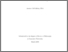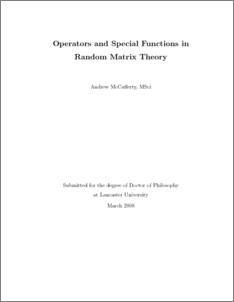# Operators and special functions in random matrix theory

McCafferty, Andrew James (2008) Operators and special functions in random matrix theory. PhD thesis, UNSPECIFIED.Preview
PDF (2008mccaffertyphd)
thesis.pdf - Published Version

## Abstract

The Fredholm determinants of integral operators with kernel of the form (A(x)B(y) − A(y)B(x))/(x−y) arise in probabilistic calculations in Random Matrix Theory. These were extensively studied by Tracy and Widom, so we refer to them as Tracy–Widom operators. We prove that the integral operator with Jacobi kernel converges in trace norm to the integral operator with Bessel kernel under a hard edge scaling, using limits derived from convergence of differential equation coefficients. The eigenvectors of an operator with kernel of Tracy–Widom type can sometimes be deduced via a commuting differential operator. We show that no such operator exists for TW integral operators acting on L2(R). There are analogous operators for discrete random matrix ensembles, and we give sufficient conditions for these to be expressed as the square of a Hankel operator: writing an operator in this way aids calculation of Fredholm determinants. We also give a new example of discrete TW operator which can be expressed as the sum of a Hankel square and a Toeplitz operator.

Item Type:
Thesis (PhD)
Uncontrolled Keywords:
/dk/atira/pure/researchoutput/libraryofcongress/qa
Subjects:
Departments:
ID Code:
13101
Deposited By:
Deposited On:
03 Oct 2008 11:25
Refereed?:
No
Published?:
Published